• +91 9971497814
• info@interviewmaterial.com

# RD Chapter 32- Statistics Ex-32.1 Interview Questions Answers

### Related Subjects

Question 1 :
Calculate the mean deviation about the median of the following observation :
(i) 3011, 2780, 3020, 2354, 3541, 4150, 5000
(ii) 38, 70, 48, 34, 42, 55, 63, 46, 54, 44
(iii) 34, 66, 30, 38, 44, 50, 40, 60, 42, 51
(iv) 22, 24, 30, 27, 29, 31, 25, 28, 41, 42
(v) 38, 70, 48, 34, 63, 42, 55, 44, 53, 47

(i) 3011, 2780, 3020, 2354, 3541, 4150, 5000
To calculate the Median (M), let us arrange the numbers in ascending order.
Median is the middle number of all the observation.
2354, 2780, 3011, 3020, 3541, 4150, 5000
So, Median = 3020 and n = 7
By using the formula to calculate Mean Deviation,
MD=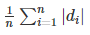xi |di| = |xi – 3020| 3011 9 2780 240 3020 0 2354 666 3541 521 4150 1130 5000 1980 Total 4546
MD== 1/7 × 4546
= 649.42
∴ The Mean Deviation is 649.42.
(ii) 38, 70, 48, 34, 42, 55, 63, 46, 54, 44
To calculate the Median (M), let us arrange the numbers in ascending order.
Median is the middle number of all the observation.
34, 38, 42, 44, 46, 48, 54, 55, 63, 70
Here the Number of observations are Even then Median = (46+48)/2 = 47
Median = 47 and n = 10
By using the formula to calculate Mean Deviation,
MD=xi |di| = |xi – 47| 38 9 70 23 48 1 34 13 42 5 55 8 63 16 46 1 54 7 44 3 Total 86

MD== 1/10 × 86
= 8.6
∴ The Mean Deviation is 8.6.
(iii) 34, 66, 30, 38, 44, 50, 40, 60, 42, 51
To calculate the Median (M), let us arrange the numbers in ascending order.
Median is the middle number of all the observation.
30, 34, 38, 40, 42, 44, 50, 51, 60, 66
Here the Number of observations are Even then Median = (42+44)/2 = 43
Median = 43 and n = 10
By using the formula to calculate Mean Deviation,
MD=xi |di| = |xi – 43| 30 13 34 9 38 5 40 3 42 1 44 1 50 7 51 8 60 17 66 23 Total 87
MD== 1/10 × 87
= 8.7
∴ The Mean Deviation is 8.7.
(iv) 22, 24, 30, 27, 29, 31, 25, 28, 41, 42
To calculate the Median (M), let us arrange the numbers in ascending order.
Median is the middle number of all the observation.
22, 24, 25, 27, 28, 29, 30, 31, 41, 42
Here the Number of observations are Even then Median = (28+29)/2 = 28.5
Median = 28.5 and n = 10
By using the formula to calculate Mean Deviation,
MD=xi |di| = |xi – 28.5| 22 6.5 24 4.5 30 1.5 27 1.5 29 0.5 31 2.5 25 3.5 28 0.5 41 12.5 42 13.5 Total 47
MD== 1/10 × 47
= 4.7
∴ The Mean Deviation is 4.7.
(v) 38, 70, 48, 34, 63, 42, 55, 44, 53, 47
To calculate the Median (M), let us arrange the numbers in ascending order.
Median is the middle number of all the observation.
34, 38, 43, 44, 47, 48, 53, 55, 63, 70
Here the Number of observations are Even then Median = (47+48)/2 = 47.5
Median = 47.5 and n = 10
By using the formula to calculate Mean Deviation,
MD=xi |di| = |xi – 47.5| 38 9.5 70 22.5 48 0.5 34 13.5 63 15.5 42 5.5 55 7.5 44 3.5 53 5.5 47 0.5 Total 84
MD== 1/10 × 84
= 8.4
∴ The Mean Deviation is 8.4.

Question 2 :
Calculate the mean deviation from the mean for the following data :
(i) 4, 7, 8, 9, 10, 12, 13, 17
(ii) 13, 17, 16, 14, 11, 13, 10, 16, 11, 18, 12, 17
(iii) 38, 70, 48, 40, 42, 55, 63, 46, 54, 44
(iv) 36, 72, 46, 42, 60, 45, 53, 46, 51, 49
(v) 57, 64, 43, 67, 49, 59, 44, 47, 61, 59

(i) 4, 7,8, 9, 10, 12, 13, 17

We know that,

MD=Where, |di| = |xi – x|

So, let ‘x’ be the mean of the given observation.

x = [4 + 7 + 8 + 9 + 10 + 12 + 13 + 17]/8

= 80/8

= 10

Number of observations, ‘n’ = 8

 xi |di| = |xi – 10| 4 6 7 3 8 2 9 1 10 0 12 2 13 3 17 7 Total 24

MD== 1/8 × 24

= 3

TheMean Deviation is 3.

(ii) 13, 17,16, 14, 11, 13, 10, 16, 11, 18, 12, 17

We know that,

MD=Where, |di| = |xi – x|

So, let ‘x’ be the mean of the given observation.

x = [13 + 17 + 16 + 14 + 11 + 13 + 10 + 16 + 11 + 18 + 12 +17]/12

= 168/12

= 14

Number of observations, ‘n’ = 12

 xi |di| = |xi – 14| 13 1 17 3 16 2 14 0 11 3 13 1 10 4 16 2 11 3 18 4 12 2 17 3 Total 28

MD== 1/12 × 28

= 2.33

TheMean Deviation is 2.33.

(iii) 38, 70,48, 40, 42, 55, 63, 46, 54, 44

We know that,

MD=Where, |di| = |xi – x|

So, let ‘x’ be the mean of the given observation.

x = [38 + 70 + 48 + 40 + 42 + 55 + 63 + 46 + 54 + 44]/10

= 500/10

= 50

Number of observations, ‘n’ = 10

 xi |di| = |xi – 50| 38 12 70 20 48 2 40 10 42 8 55 5 63 13 46 4 54 4 44 6 Total 84

MD== 1/10 × 84

= 8.4

TheMean Deviation is 8.4.

(iv) 36, 72,46, 42, 60, 45, 53, 46, 51, 49

We know that,

MD=Where, |di| = |xi – x|

So, let ‘x’ be the mean of the given observation.

x = [36 + 72 + 46 + 42 + 60 + 45 + 53 + 46 + 51 + 49]/10

= 500/10

= 50

Number of observations, ‘n’ = 10

 xi |di| = |xi – 50| 36 14 72 22 46 4 42 8 60 10 45 5 53 3 46 4 51 1 49 1 Total 72

MD== 1/10 × 72

= 7.2

TheMean Deviation is 7.2.

(v) 57, 64,43, 67, 49, 59, 44, 47, 61, 59

We know that,

MD=Where, |di| = |xi – x|

So, let ‘x’ be the mean of the given observation.

x = [57 + 64 + 43 + 67 + 49 + 59 + 44 + 47 + 61 + 59]/10

= 550/10

= 55

Number of observations, ‘n’ = 10

 xi |di| = |xi – 55| 57 2 64 9 43 12 67 12 49 6 59 4 44 11 47 8 61 6 59 4 Total 74

MD== 1/10 × 74

= 7.4

TheMean Deviation is 7.4.

Question 3 :

Calculate the meandeviation of the following income groups of five and seven members from theirmedians:

 I Income in ₹ II Income in ₹ 4000 3800 4200 4000 4400 4200 4600 4400 4800 4600 4800 5800

Let us calculate the mean deviation for the first data set.

Since the data is arranged in ascending order,

4000, 4200, 4400, 4600, 4800

Median = 4400

Total observations = 5

We know that,

MD=Where, |di| = |xi – M|

 xi |di| = |xi – 4400| 4000 400 4200 200 4400 0 4600 200 4800 400 Total 1200

MD== 1/5 × 1200

= 240

Let us calculate the mean deviation for the second data set.

Since the data is arranged in ascending order,

3800, 4000, 4200, 4400, 4600, 4800, 5800

Median = 4400

Total observations = 7

We know that,

MD=Where, |di| = |xi – M|

 xi |di| = |xi – 4400| 3800 600 4000 400 4200 200 4400 0 4600 200 4800 400 5800 1400 Total 3200

MD== 1/7 × 3200

= 457.14

TheMean Deviation of set 1 is 240 and set 2 is 457.14

Question 4 :
The lengths (in cm) of 10 rods in a shop are given below:
40.0, 52.3, 55.2, 72.9, 52.8, 79.0, 32.5, 15.2, 27.9, 30.2
(i) Find the mean deviation from the median.
(ii) Find the mean deviation from the mean also.

(i) Findthe mean deviation from the median

Let us arrange the data in ascending order,

15.2, 27.9, 30.2, 32.5, 40.0, 52.3, 52.8, 55.2, 72.9, 79.0

We know that,

MD=Where, |di| = |xi – M|

The number of observations are Even then Median =(40+52.3)/2 = 46.15

Median = 46.15

Number of observations, ‘n’ = 10

 xi |di| = |xi – 46.15| 40.0 6.15 52.3 6.15 55.2 9.05 72.9 26.75 52.8 6.65 79.0 32.85 32.5 13.65 15.2 30.95 27.9 19.25 30.2 15.95 Total 167.4

MD== 1/10 × 167.4

= 16.74

TheMean Deviation is 16.74.

(ii) Findthe mean deviation from the mean also.

We know that,

MD=Where, |di| = |xi – x|

So, let ‘x’ be the mean of the given observation.

x = [40.0 + 52.3 + 55.2 + 72.9 + 52.8 + 79.0 + 32.5 + 15.2 +27.9 + 30.2]/10

= 458/10

= 45.8

Number of observations, ‘n’ = 10

 xi |di| = |xi – 45.8| 40.0 5.8 52.3 6.5 55.2 9.4 72.9 27.1 52.8 7 79.0 33.2 32.5 13.3 15.2 30.6 27.9 17.9 30.2 15.6 Total 166.4

MD== 1/10 × 166.4

= 16.64

The Mean Deviation is 16.64

Question 5 : In question 1(iii), (iv), (v) find the number of observations lying between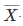–M.D and+M.D, where M.D. is the mean deviation from the mean.

(iii) 34,66, 30, 38, 44, 50, 40, 60, 42, 51

We know that,

MD=Where, |di| = |xi – x|

So, let ‘x’ be the mean of the given observation.

x = [34 + 66 + 30 + 38 + 44 + 50 + 40 + 60 + 42 + 51]/10

= 455/10

= 45.5

Number of observations, ‘n’ = 10

 xi |di| = |xi – 45.5| 34 11.5 66 20.5 30 15.5 38 7.5 44 1.5 50 4.5 40 5.5 60 14.5 42 3.5 51 5.5 Total 90

MD== 1/10 × 90

= 9

NowM.D = 45.5 – 9 = 36.5+M.D = 45.5 + 9 = 54.5

So,There are total 6 observation betweenM.D and+M.D

(iv) 22, 24,30, 27, 29, 31, 25, 28, 41, 42

We know that,

MD=Where, |di| = |xi – x|

So, let ‘x’ be the mean of the given observation.

x = [22 + 24 + 30 + 27 + 29 + 31 + 25 + 28 + 41 + 42]/10

= 299/10

= 29.9

Number of observations, ‘n’ = 10

 xi |di| = |xi – 29.9| 22 7.9 24 5.9 30 0.1 27 2.9 29 0.9 31 1.1 25 4.9 28 1.9 41 11.1 42 12.1 Total 48.8

MD== 1/10 × 48.8

= 4.88

Now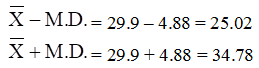So, There are total 5 observation between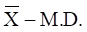and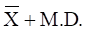(v) 38, 70,48, 34, 63, 42, 55, 44, 53, 47

We know that,

MD=Where, |di| = |xi – x|

So, let ‘x’ be the mean of the given observation.

x = [38 + 70 + 48 + 34 + 63 + 42 + 55 + 44 + 53 + 47]/10

= 494/10

= 49.4

Number of observations, ‘n’ = 10

 xi |di| = |xi – 49.4| 38 11.4 70 20.6 48 1.4 34 15.4 63 13.6 42 7.4 55 5.6 44 5.4 53 3.6 47 2.4 Total 86.8

MD== 1/10 × 86.8

= 8.68

Now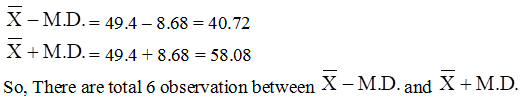Todays Deals### RD Chapter 32- Statistics Ex-32.1 Contributorskrishan

Name:
Email:

# Latest News# 9000 interview questions in different categories